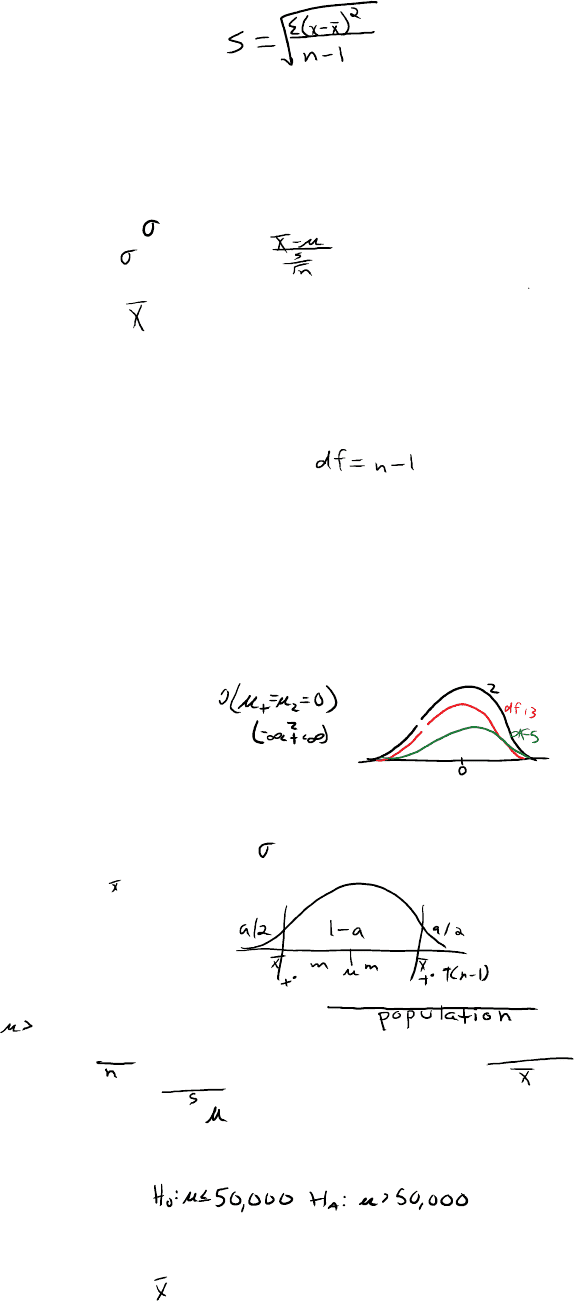# BUSMGT 2320 Lecture 4: 4. Lecture 4 - One Sample T; Paired Samples T

44 views4 pages

For unlimited access to Class Notes, a Class+ subscription is required.t
Test statistic
t , t , t
Critical Value (Point)
Matched (paired) samples
Notation:
Estimate with s, giving
No longer Standard Normal Z, no longer Z-scores
Assuming is Normally distributed, result is now a t random variable
Still measures # of standard deviations from mean
Substituting s for : Student's t
In the one-sample case
df = # of observation used to calculate sum of squared deviations - \$ of
mathematical restrictions
There is a family of t-curves
Has one parameter: degrees of freedom: df, DF
The t-Distribution
Same symmetric mound shape
Same center,
Both asymptotic
t-curves have fatter tails
t-curves become taller and skinnier as df increase
Observations:
Comparing t to Z
m = t*s
Symmetric case:
Reasonable Sampling Error - Unknown
PROBLEM: average income of households within 25 miles must exceed
\$50,000 for success
SRS of 64 and found average annual income of \$50,725 with standard
deviation of \$4200
Does this indicate > \$50,000
PLAN
Hypothesis:
SRS
s = \$4200
n = 64; income is usually skewed, but CLT probably applies, given n > 2(30)
n = <.1N SE( ) = no adjustment
The Unlimited Avenue Case
Lecture 4
-
One sample t; Paired Samples t
Thursday, February 2, 2017 06:59 PM
Unit 1 Page 1
Unlock document

This preview shows page 1 of the document.
Unlock all 4 pages and 3 million more documents.

Already have an account? Log in

# Get access

Grade+
\$10 USD/m
Billed \$120 USD annually
Homework Help
Class Notes
Textbook Notes
40 Verified Answers
Study Guides
1 Booster Class
Class+
\$8 USD/m
Billed \$96 USD annually
Homework Help
Class Notes
Textbook Notes
30 Verified Answers
Study Guides
1 Booster Class